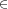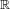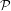## Chapter 13Notes for the third week: set theory

Notes also available as PDF.

### 13.1 Language of set theory

• We will cover just enough set theory to use later.
• Cardinalities are important for probability. We don’t have time to cover probability sufficiently well, so we will not explore the sizes of sets deeply.
• This is known as naïve set theory. We do not define absolutely everything, nor do we push set theory’s logical limits. Much.

Goals:

• Impart some of the language necessary for later chapters.
• Practice reasoning in a formal setting.
• One key aspect is what to do in extreme cases like empty sets.
• Set up straight-forward examples for logic.

### 13.2 Basic definitions

To start, we require unambiguous definitions of terms and items. When a term or item is unambiguously defined, it is called well-defined.

set
An unordered collection of unique elements.
• Curly braces: \{A,B,C\} is a set of three elements, A, B, and C.
• Order does not matter: \{\text{cat},\text{dog}\} is the same set as \{\text{dog},\text{cat}\}.
• Repeated elements do not matter: \{1,1,1\} is the same set as \{1\}.
• Can be implicit: \{x\kern 1.66702pt |\kern 1.66702pt x\text{ is an integer},x > 0,x < 3\} is the same set as \{1,2\}.
• Read the implicit form as “the set of elements x such that x is an integer, x > 0, and x < 3”. Or “the set of elements x where \mathop{\mathop{…}}
• Other symbols that sometimes stand for “such that”: :, (reversed)
• Implicit (or set-builder) form can include formula or other bits left of the bar. \{3x\kern 1.66702pt |\kern 1.66702pt x\text{ is a positive integer}\} is the set \{3,6,9,\mathop{\mathop{…}}\}.
element
Any item in a set, even other sets. (Also entry, member, item, etc.)
• This is not ambiguous. If something is in a set, it is an item of that set. It doesn’t matter if the item is a number or a grape.
• \{A,\{B,C\}\} is a set of two elements, A and \{B,C\}.
• None of the following are the same: \{A,\{B,C\}\}, \{A,B,C\}, \{\{A,B\},C\}.
empty set
Or null set. Denoted by rather than \{\}.
• This is a set on its own.
• \{∅\} is the set of the empty set, which is not empty.
• Think of sets as bags. An empty bag still is a bag, and if a bag contains an empty bag, the outer bag is not empty.
• Implicit definitions can hide empty sets.
• For example, the set \{x\kern 1.66702pt |\kern 1.66702pt x\text{ is an odd integer divisible by }2\} is .
singleton
A set with only one element.
• \{1\} and \{∅\} both are singletons (or sometimes singleton sets).

### 13.3 Translating sets into (and from) English

From English:

• The days of the week:
• \{\text{Monday},\text{Tuesday},\text{Wednesday},\text{Thursday},\text{Friday},\text{Saturday},\text{Sunday}\}
• Of course, we’re using a representation of the days and not the days themselves. That is how we reason about things; we model them and represent them by symbols.
• The days when homework is due:
• \{\text{25th of August},\text{1st of September},\mathop{\mathop{…}}\}
• We could list them all.
• \{ every Monday after the 18th of August 2008 until after the 1st of December \}
• Or: \{x\kern 1.66702pt |\kern 1.66702pt x is a Monday, x is after the 18th of August, and x is on or before the 1st of December \}

To English:

• \{2,3,4\}:
• The set containing two, three, and four.
• \{x\kern 1.66702pt |\kern 1.66702pt x\text{ is an integer and }x > 0\}:
• The positive integers, also called the counting numbers or the natural numbers.
• Often written as {J}^{+}. The integers often are written as J (because the “I” form can be difficult to read), rationals as (for quotients), the reals as.
• \{2x - 1\kern 1.66702pt |\kern 1.66702pt x{J}^{+}\}
• The set whose members have the form 2x - 1 where x is a positive integer.
• Cannot list all the entries; this is an infinite set.
• Here, the odd integers.

### 13.4 Relations

element of
The expression xA states that x is an element of A. If x\mathrel{∉}A, then x is not an element of A.
• 4\{2,4,6\}, and 4\mathrel{∉}\{x\kern 1.66702pt |\kern 1.66702pt x\text{ is an odd integer }\}.
• There is no x such that x, so \{x\kern 1.66702pt |\kern 1.66702pt x∅\} is a long way of writing .
subset
If all entries of set A also are in set B, A is a subset of B.
superset
The reverse of subset. If all entries of set B also are in set A, then A is a superset of B.
proper subset
If all entries of set A also are in set B, but some entries of B are not in A, then A is a proper subset of B.
• \{2,3\} is a proper subset of \{1,2,3,4\}.
equality
Set A equals set B when A is a subset of B and B is a subset of A.
• Order does not matter. \{1,2,3\} = \{3,2,1\}.

The symbols for these relations are subject to a little disagreement.

• Many basic textbooks write the subset relation as , so A ⊆ B when A is a subset of B. The same textbooks reserve for the proper subset. Supersets are .
• This keeps a superficial similarity to the numerical relations and <. In the former the compared quantities may be equal, while in the latter they must be different.
• Most mathematicians now use for any subset. If a property requires a “proper subset”, it often is worth noting specifically. And the only non-“proper subset” of a set is the set itself.
• Extra relations are given for emphasis, e.g. or for proper subsets and or to emphasize the possibility of equality.
• Often a proper subset is written out: A ⊂ B and A≠B.
• I’ll never remember to stick with the textbook’s notation. My use of \mathbf{⊂} is for subsets and not proper subsets.

### 13.5 Translating relations into (and from) English

From English:

• The train has a caboose.
• It’s reasonable to think of a train as a set of cars (they can be reordered).
• The cars are the members.
• Hence, caboosetrain
• The VI volleyball team consists of VI students.
• VI volleyball team VI students
• There are no pink elephants.
• pink elephants = ∅

To English:

• xtoday’s homework set.
• x is a problem in today’s homework set.
• Today’s homework this week’s homework.
• Today’s homework is a subset of this week’s homework.

### 13.6 Consequences of the set relation definitions

Every set is a subset of itself. Expected.

If A = B, then every member of A is a member of B, and every member of B is a member of A. This is what we expect from equality, but we did not define set equality this way. Follow the rules:

• A = B imples A ⊂ B and B ⊂ A.
• Because A ⊂ B, every member of A is a member of B.
• Because B ⊂ A, every member of B is a member of A.

The empty set is a subset of all sets. Unexpected! This is a case of carrying the formal logic to its only consistent end.

• For some set A, ∅⊂ A if every member of is in A.
• But has no members.
• Thus all of ’s members also are in A.
• This is called a vacuous truth.

The alternatives would not be consistent, but proving that requires more machinery that we need.

### 13.7 Visualizing two or three sets: Venn diagrams

Also known as Venn diagrams.

yes, at some point I will draw some and stick them in the notes.

### 13.8 Operations

union
The union of two sets A and B, denoted by A ∪ B, is the set consisting of all elements from A and B.
• A ∪ B = \{x\kern 1.66702pt |\kern 1.66702pt xA\text{ or }xB\}.
• Remember repeated elements do not matter: \{1,2\} ∪\{2,3\} = \{1,2,3\}.
intersection
The intersection of two sets A and B, denoted A ∩ B, is the set consisting of all elements that are in both A and B.
• A ∩ B = \{x\kern 1.66702pt |\kern 1.66702pt xA\text{ and }xB\}.
• \{1,2\} ∩\{2,3\} = \{2\}.
• \{1,2\} ∩\{3,4\} = \{\} = ∅.
set difference
The set difference of two sets A and B, written A \ B, is the set of entries of A that are not entries of B.
• A \ B = \{x\kern 1.66702pt |\kern 1.66702pt xA\text{ and }x∉B\}.
• Sometimes written as A - B, but that often becomes confusing.

If A and B share no entries, they are called disjoint. One surprising consequence is that every set A has a subset disjoint to the set A itself.

• No sets (not even ) can share elements with because has no elements.
• So all sets are disjoint with .
• The empty set is a subset of all sets.
• So all sets are disjoint with at least one of their subsets!

Can any other subset be disjoint with its superset? No.

#### 13.8.1 Similarities to arithmetic

Properties of arithmetic:

commutative
a + b = b + a, a ⋅ b = b ⋅ a
associative
a + (b + c) = (a + b) + c, a(bc) = (ab)c
distributive
a(b + c) = ab + ac

Which of these apply to set operations union and intersection? (Informally. Formally we must rely on the properties of and and or.)

If C = A ∪ B, then C = \{x\kern 1.66702pt |\kern 1.66702pt xA\text{ or }xB\}. Reversing the sets does not matter, so C = A ∪ B = B ∪ A. The union is commutative. Similary, if D = A ∪ (B ∪ C), we can write D in an implicit form and see that D = (A ∪ B) ∪ C to see that the union is associative.

The same arguments show that set intersection is commutative and associative.

For the distributive property, which is similar to addition and which to multiplication? A gut feeling is that unions add, so try it.

\eqalignno{ A ∩ (B ∪ C) & = \{x\kern 1.66702pt |\kern 1.66702pt xA\text{ and }xB ∪ C\} & & \cr & = \{x\kern 1.66702pt |\kern 1.66702pt xA\text{ and }(xB\text{ or }xC)\} & & \cr & = \{x\kern 1.66702pt |\kern 1.66702pt (xA\text{ and }xB)\text{ or }(xA\text{ and }xC)\} & & \cr & = (A ∩ B) ∪ (A ∩ C) & & }

But with sets, both operations distribute:

\eqalignno{ A ∪ (B ∩ C) & = \{x\kern 1.66702pt |\kern 1.66702pt xA\text{ or }xB ∩ C\} & & \cr & = \{x\kern 1.66702pt |\kern 1.66702pt xA\text{ or }(xB\text{ and }xC)\} & & \cr & = \{x\kern 1.66702pt |\kern 1.66702pt (xA\text{ or }xB)\text{ and }(xA\text{ or }xC)\} & & \cr & = (A ∪ B) ∩ (A ∪ C) & & }

The rules of set theory are intimately tied to logic. Logical operations dictate how set operations behave. We will cover the properties of logic in the next chapter.

### 13.9 Translating operations into English

To English:

• (A ∪ B) ∩ C
• The set consisting of members that are in C and either of A or B.
• (A ∩ B) ∪ C
• The set consisting of members that are in C or in both of A or B.

### 13.10 Special operations

The complement and cross-product operations require extra definitions.

#### 13.10.1 Universes and complements

universe
A master set containing all the other sets in the current context.
complement
The complement of a set A is the set of all elements in a specified universal set U that are not in A.
• {A}^{c} = \{x\kern 1.66702pt |\kern 1.66702pt x∉A\text{ and }xU\} = U - A.
• Sometimes written as A' or \bar{A}.
• It’s not always necessary to define a universal set.
• And there is no “universal” universal set.
• Because {A}^{c} = U \ A, many people avoid the complement completely.
• The complement is useful to avoid writing many repeated U \ A operations that share the same universal set.

#### 13.10.2 Tuples and cross products

tuple
An ordered collection of elements, (A,B,C).
• When only two elements, this is an ordered pair.
• Think of coordinates in a graph, (x,y).
• So (x,y)≠(y,x) in general (i.e. when x≠y).
cross product
A set of all ordered pairs whose entries are drawn from two sets.
• A × B = \{(x,y)\kern 1.66702pt |\kern 1.66702pt xA,yB\}.

Let A = \{{a}_{1},{a}_{2}\} and B = \{{b}_{1},{b}_{2}\}.

Then A × B = \{({a}_{1},{b}_{1}),({a}_{1},{b}_{2}),({a}_{2},{b}_{1}),({a}_{2},{b}_{2})\} and
B × A = \{({b}_{1},{a}_{1}),({b}_{1},{a}_{2}),({b}_{2},{a}_{1}),({b}_{2},{a}_{2})\}. Because ({a}_{1},{b}_{1})≠({b}_{1},{a}_{1}) in general, A × B≠B × A in general.

When does A × B = B × A?

### 13.11 Cardinality and the power set

cardinality
The cardinality of a set A is the number of elements in A. Often written as |A|. The text uses n(A).
• If A = \{1,2,3\}, then |A| = 3.
• What is |∅|? 0.
power set
The power set of a set A is the set of all subsets of A.
• Often denoted as(A), but this is used rarely enough that the notation always needs defined.

What is the cardinality of the power set of A?

• What is cardinality of the power set of ?
• All sets are subsets of themselves, and the empty set is a subset of itself.
• Then(∅) = \{∅\}, and || = |\{∅\}| = 1.
• What is the powerset of a set with one element, let’s say \{1\}?
• There are two subsets, and the set itself \{1\}.
•(\{1\}) = \{∅,\{1\}\}, and |(\{1\})| = 2.
• Two elements, say \{1,2\}?
•(\{1,2\}) = \{∅,\{1\},\{2\},\{1,2\}\}.
• |(\{1,2\})| = 4.
• So the powerset with zero entries has size 1, one entry has size 2, two has size 4, \mathop{\mathop{…}}

What is the cardinality of A ∪ B?

• Sets do not contain repeated members, so the union cannot be simply the sum of its arguments.
• The intersection contains one copy of all the shared members.
• So to count every item once the cardinality of the union is the sum of the cardinalities of the sets minus the cardinality of the intersection.
• |A ∪ B| = |A| + |B|-|A ∩ B|.
• Known as the inclusion-exclusion principle.
• Extends to more sets, but you must be careful about counting entries once!
• |A ∪ B ∪ C| = |A| + |B| + |C|-|A ∩ B|-|B ∩ C|-|A ∩ C| + |A ∩ B ∩ C|.